## How to use a Scientific Calculator

Learning to use a scientific calculator is essential to help you with your exams. Most exam boards have at least one paper where you will be required to use a calculator.

You will need to familiarise yourself with your scientific calculator and learn the uses and functions of many of the buttons. Not all scientific calculators contain all the buttons, so you will need to know how to use substitutions if applicable.

Some of the main buttons you will need to use are highlighted below. With each example below we have highlighted the order the buttons should be pressed on your calculator.

Square Button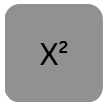Example 12²: 12 x² = 144

Cube Button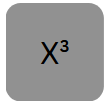Example 8³: 8 x³ = 512

Power Button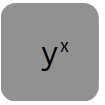Example 4⁵: 4 yx 5 = 1024

Square Root Button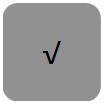Example √40: 40 √ = 6.32

Cube Root Button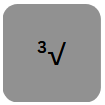Example ³√125: 2nd F ³√ 125 = 5

xth Root Button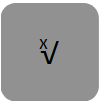Example ⁵√32: 5 2nd F x32 = 2

Fraction Button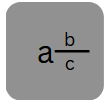Example 8: 8 a b 5 = 1 3
5         c           5

Standard Form Button (Some calculators will use a button with EXP)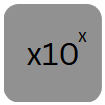Example 3.4 x 10⁴: 3.4  x10x 4 = 34 000

Using Brackets on a Calculator

Large calculations will usually require you to use brackets when using your calculator.

Example Calculate 15 + 3.5²
7.6 – 1.6

Type ( 15 + 3.5 x² ) ÷ ( 7.6 – 1.6 ) = 4.541

Using a Calculator to work out Standard Form

Brackets are essential to separate the numbers when calculating standard form on a calculator.

Here is an example:  (3.3 x 10⁴) x (3.2 x 10⁵)

Type the following in the calculator ( 3.3 x10x 4 ) x (3.2 x10x 5 ) = 1.056 1010

The video below shows you how to calculate standard form with or without a calculator.